# OpenCV | Understanding Contrast in an Image

In OpenCV, changing the contrast of an image is a very basic task to perform. By changing the image contrast, it is meant to change the value of each and every image pixel. This change can be done by either multiplying or dividing (means to multiply each pixel with value < 1) the pixel values of the image, by any constant.

To increase the contrast levels of the image, simply multiply a constant positive value to each and every image pixel.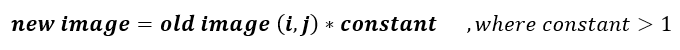Similarly if one wishes to decrease the contrast level of the image, then multiply a constant positive value less than 1 for each and every image pixel.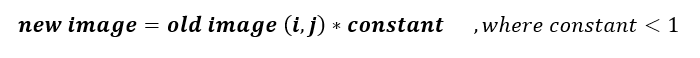Let’s take an assumption by considering the below-mentioned matrix as our image matrix with values of pixel ranging from 0 to 255 and let’s assume that the data type for this image is CV_8UC3.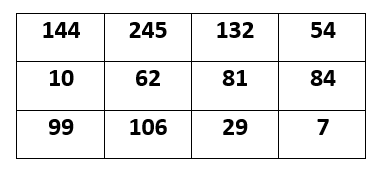## Increasing contrast :

So. let’s suppose we wish to increase image contrast by a factor of 2. Then we multiply 2 to each image pixel value but it is strictly forbidden to exceed the pixel range i.e. the allowed maximum limit is 255 and our pixel value should not increase 255. Suppose if in any case, this image pixel value exceeds 255, then it should be given the maximum allowed value only instead of the newly increased value.

Let’s check the image matrix given below, we have multiply this value by factor of 2 but the pixel value “255” has not been increased to 288 as we multiply 2 to 144, it should be 288 but this is not happening here because the maximum image pixel value is allowed to be 255 in this image.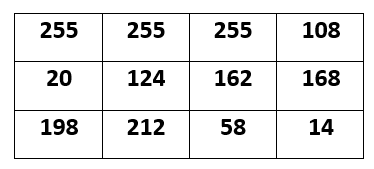## Decreasing contrast :

Here, we are decreasing the contrast values by a factor of 0.5 and to do so 0.5 is multiplied to each and every image pixel in the input image but the only thing important is that the value of any pixel should not go below the minimum allowed pixel.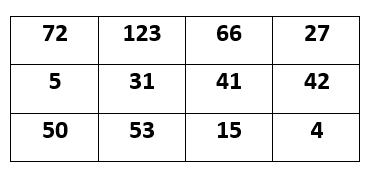As here in our output image, the contrast is decreased by a factor of 0.5.

Don’t stop now and take your learning to the next level. Learn all the important concepts of Data Structures and Algorithms with the help of the most trusted course: DSA Self Paced. Become industry ready at a student-friendly price.

My Personal Notes arrow_drop_upCheck out this Author's contributed articles.

If you like GeeksforGeeks and would like to contribute, you can also write an article using contribute.geeksforgeeks.org or mail your article to contribute@geeksforgeeks.org. See your article appearing on the GeeksforGeeks main page and help other Geeks.

Please Improve this article if you find anything incorrect by clicking on the "Improve Article" button below.

Article Tags :
Practice Tags :

Be the First to upvote.

Please write to us at contribute@geeksforgeeks.org to report any issue with the above content.